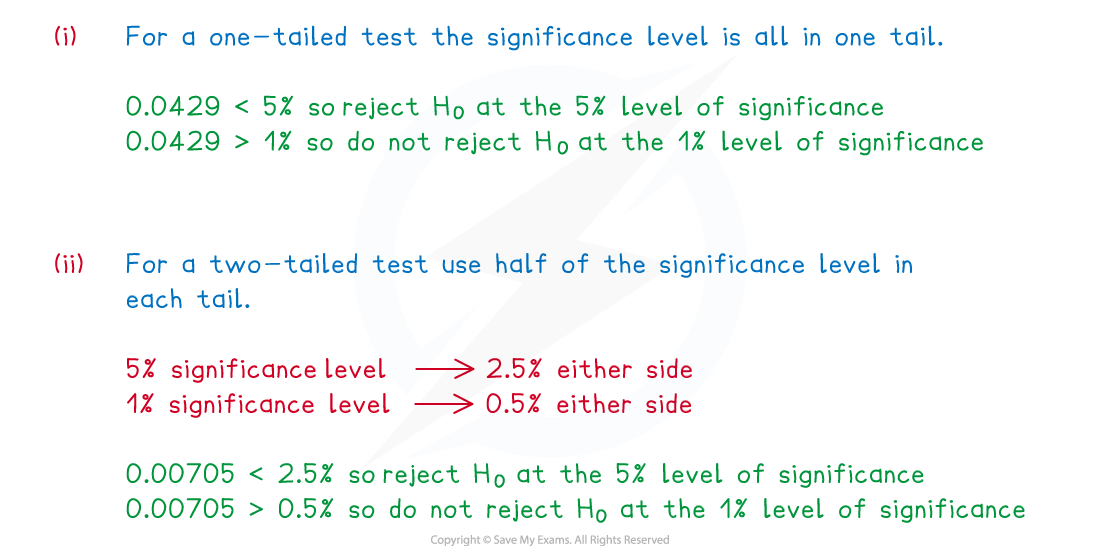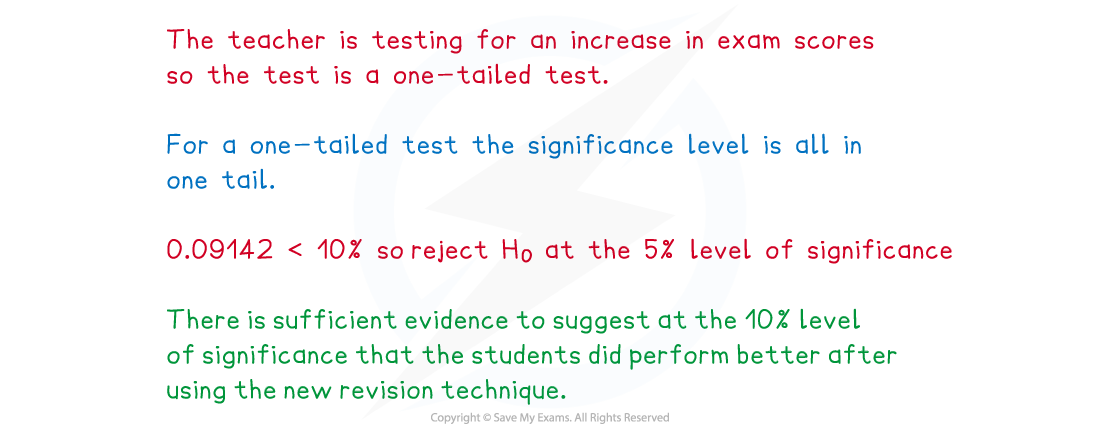# AQA A Level Maths: Statistics复习笔记5.1.1 Hypothesis Testing

### Language of Hypothesis Testing

#### What is a hypothesis test?

• A hypothesis test uses a sample of data in an experiment to test a statement made about the value of a population parameter
• A hypothesis test is used when the value of the assumed population parameter is questioned
• The hypothesis test will look at the which outcomes are unlikely to occur if assumed population parameter is true
• The probability found will be compared against a given significance level to determine whether there is evidence to believe that the assumed population parameter is not true

#### What are the key terms used in statistical hypothesis testing?

• Every hypothesis test must begin with a clear null hypothesis (what we believe to already be true) and alternative hypothesis (how we believe the data pattern or probability distribution might have changed)
• A hypothesis is an assumption that is made about a particular population parameter
• A population parameter is a numerical characteristic which helps define a population
• One example of a population parameter is the probability, p  of an event occurring
• Another example is the mean of a population
• The null hypothesis is denoted H0 and sets out the assumed population parameter given that no change has happened
• The alternative hypothesis is denoted H1  and sets out how we think the population parameter could have changed
• When a hypothesis test is carried out, the null hypothesis is assumed to be true and this assumption will either be accepted or rejected
• A hypothesis test could be a one-tailed test or a two-tailed test
• The null hypothesis will always be H0 : θ = ...
• The alternate hypothesis will depend on if it is a one-tailed or two-tailed test
• A one-tailed test would test to see if the population parameter, θ, has either increased or decreased
• The alternative hypothesis, H1  will be H1 : θ > ...  or  H1 : θ < ...
• A two-tailed test would test to see if the population parameter, θ , has changed
• The alternative hypothesis, H1  will be H1 : θ ≠ ...
• It is important to read the wording of the question carefully to decide whether your hypothesis test should be one-tailed or two-tailed
• To carry out a hypothesis test an experiment will be carried out on a sample of data, the result of this experiment will be the test statistic
• A sample of data is a subset of data taken from the population
• The test statistic is a numerical value calculated from the of data
• A hypothesis test will always be carried out at an appropriate significance level
• The significance level sets the smallest probability that an event could have occurred by chance.
• Any probability smaller than the significance level would suggest that the event is unlikely to have happened by chance
• The significance level must be set before the hypothesis test is carried out
• The significance level will usually be 1%, 5% or 10%, however it may vary

#### Worked Example

A hypothesis test is carried out at the 5% level of significance to test if a normal coin is fair or not.

(i)Describe what the population parameter could be for the hypothesis test.
(ii)State whether the hypothesis test should be a one-tailed test or a two-tailed test, give a reason for your answer.
(iii)Clearly defining your population parameter, state suitable null and alternative hypotheses for the test.#### Exam Tip

• Make sure you read the question carefully to determine whether the test you are carrying out is for a one-tailed or a two-tailed test and use the level of significance accordingly.

### Critical Regions & p-values

#### How do we decide whether to reject or accept the null hypothesis?

• The null hypothesis would be rejected if the p - value is less than the significance level or if the test statistic falls within the critical region
• The p - value is the probability of a value being at least as extreme as the test statistic, assuming that the null hypothesis is true
• If the test is looking for a decrease then extreme values are smaller than the test statistic, so find the probability of less than or equal to the test statistic
• If the test is looking for an increase then extreme values are bigger than the test statistic, so find the probability of greater than or equal to the test statistic
• In a two-tailed test the p - value is double this probability
• Though for a two-tailed test it is common to half the significance level and compare this with the probability (rather than doubling the probability)
• The critical region is the range of values of the test statistic which will lead to the null hypothesis being rejected
• If the test statistic falls within the critical region, the null hypothesis would be rejected
• The critical value is the boundary of the critical region
• It is the least extreme value that would lead to the rejection of the null hypothesis
• The critical value is determined by the significance level
• In a two-tailed test the significance level is halved and both the upper and the lower tails are tested
• For discrete distributions the critical value is the first value that falls within the critical region and so the probability of the observed value falling within the critical region may be lower than the given significance level
• This probability will be known as the actual significance level
• The actual significance level is the probability of incorrectly rejecting the null hypothesis
• Finding the critical region will be different for a two-tailed test than it is for a one-tailed test

####Worked Example

For the following situations, state at the 1% and 5% significance levels whether the null hypothesis should be rejected or not.

(i)A p–value of 0.0429 is calculated in a one-tailed hypothesis test.
(ii)Assuming the null hypothesis is true, the probability of a value being at least as extreme as the test statistic in a two-tailed hypothesis test is 0.00705.### Conclusions of Hypothesis Testing

#### How is a hypothesis test carried out?

• There are a number of ways that a hypothesis test can be carried out for different models, however the following steps should form the base for your test:
• Step 1. Define the test statistic and population parameter
• Step 2. Write the null and alternative hypotheses clearly
• Step 3. Calculate the critical value(s) or the p - value for the test
• Step 4. Compare the observed value of the test statistic with the critical value(s) or the p - value with the significance level
• Step 5. Decide whether there is enough evidence to reject H0 or whether it has to be accepted
•  Step 6. Write a conclusion in context

#### How should a conclusion be written for a hypothesis test?

• Your conclusion must be written in the context of the question
• Use the wording in the question to help you write your conclusion
• If rejecting the null hypothesis your conclusion should state that there is sufficient evidence to suggest the alternative hypothesis is true at this level of significance
• If accepting the null hypothesis your conclusion should state that there is not enough evidence to suggest the alternative hypothesis is true at this level of significance
• Your conclusion must not be definitive
• There is a chance that the test has led to an incorrect conclusion
• The outcome is dependent on the sample, a different sample might lead to a different outcome
• The conclusion of a two-tailed test can state if there is evidence of a change
• You should not state whether this change is an increase or decrease

#### Worked Example

A teacher carried out a hypothesis test at the 10% significance level to test if her students perform better in exams after using a new revision technique. The p – value for her test statistic is 0.09142. Write a conclusion for her hypothesis test.#### Exam Tip

• It is best to use the exact wording from the question when writing your conclusion for the hypothesis test, do not be afraid to sound repetitive.